Intermediate Algebra 2e

# 5.4Dividing Polynomials

Intermediate Algebra 2e5.4 Dividing Polynomials

### Learning Objectives

By the end of this section, you will be able to:

• Dividing monomials
• Dividing a polynomial by a monomial
• Dividing polynomials using long division
• Dividing polynomials using synthetic division
• Dividing polynomial functions
• Use the remainder and factor theorems

### Be Prepared 5.10

Before you get started, take this readiness quiz.

Add: $3d+xd.3d+xd.$
If you missed this problem, review Example 1.28.

### Be Prepared 5.11

Simplify: $30xy35xy.30xy35xy.$
If you missed this problem, review Example 1.25.

### Be Prepared 5.12

Combine like terms: $8a2+12a+1+3a2−5a+4.8a2+12a+1+3a2−5a+4.$
If you missed this problem, review Example 1.7.

### Dividing Monomials

We are now familiar with all the properties of exponents and used them to multiply polynomials. Next, we’ll use these properties to divide monomials and polynomials.

### Example 5.36

Find the quotient: $54a2b3÷(−6ab5).54a2b3÷(−6ab5).$

### Try It 5.71

Find the quotient: $−72a7b3÷(8a12b4).−72a7b3÷(8a12b4).$

### Try It 5.72

Find the quotient: $−63c8d3÷(7c12d2).−63c8d3÷(7c12d2).$

Once you become familiar with the process and have practiced it step by step several times, you may be able to simplify a fraction in one step.

### Example 5.37

Find the quotient: $14x7y1221x11y6.14x7y1221x11y6.$

### Try It 5.73

Find the quotient: $28x5y1449x9y12.28x5y1449x9y12.$

### Try It 5.74

Find the quotient: $30m5n1148m10n14.30m5n1148m10n14.$

### Divide a Polynomial by a Monomial

Now that we know how to divide a monomial by a monomial, the next procedure is to divide a polynomial of two or more terms by a monomial.

The method we’ll use to divide a polynomial by a monomial is based on the properties of fraction addition. So we’ll start with an example to review fraction addition. The sum $y5+25y5+25$ simplifies to $y+25.y+25.$

Now we will do this in reverse to split a single fraction into separate fractions. For example, $y+25y+25$ can be written $y5+25.y5+25.$

This is the “reverse” of fraction addition and it states that if a, b, and c are numbers where $c≠0,c≠0,$ then $a+bc=ac+bc.a+bc=ac+bc.$ We will use this to divide polynomials by monomials.

### Division of a Polynomial by a Monomial

To divide a polynomial by a monomial, divide each term of the polynomial by the monomial.

### Example 5.38

Find the quotient: $(18x3y−36xy2)÷(−3xy).(18x3y−36xy2)÷(−3xy).$

### Try It 5.75

Find the quotient: $(32a2b−16ab2)÷(−8ab).(32a2b−16ab2)÷(−8ab).$

### Try It 5.76

Find the quotient: $(−48a8b4−36a6b5)÷(−6a3b3).(−48a8b4−36a6b5)÷(−6a3b3).$

### Divide Polynomials Using Long Division

Divide a polynomial by a binomial, we follow a procedure very similar to long division of numbers. So let’s look carefully the steps we take when we divide a 3-digit number, 875, by a 2-digit number, 25.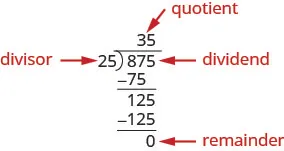We check division by multiplying the quotient by the divisor.

If we did the division correctly, the product should equal the dividend.

$35·25875✓35·25875✓$

Now we will divide a trinomial by a binomial. As you read through the example, notice how similar the steps are to the numerical example above.

### Example 5.39

Find the quotient: $(x2+9x+20)÷(x+5).(x2+9x+20)÷(x+5).$

### Try It 5.77

Find the quotient: $(y2+10y+21)÷(y+3).(y2+10y+21)÷(y+3).$

### Try It 5.78

Find the quotient: $(m2+9m+20)÷(m+4).(m2+9m+20)÷(m+4).$

When we divided 875 by 25, we had no remainder. But sometimes division of numbers does leave a remainder. The same is true when we divide polynomials. In the next example, we’ll have a division that leaves a remainder. We write the remainder as a fraction with the divisor as the denominator.

Look back at the dividends in previous examples. The terms were written in descending order of degrees, and there were no missing degrees. The dividend in this example will be $x4−x2+5x−6.x4−x2+5x−6.$ It is missing an $x3x3$ term. We will add in $0x30x3$ as a placeholder.

### Example 5.40

Find the quotient: $(x4−x2+5x−6)÷(x+2).(x4−x2+5x−6)÷(x+2).$

### Try It 5.79

Find the quotient: $(x4−7x2+7x+6)÷(x+3).(x4−7x2+7x+6)÷(x+3).$

### Try It 5.80

Find the quotient: $(x4−11x2−7x−6)÷(x+3).(x4−11x2−7x−6)÷(x+3).$

In the next example, we will divide by $2a+3.2a+3.$ As we divide, we will have to consider the constants as well as the variables.

### Example 5.41

Find the quotient: $(8a3+27)÷(2a+3).(8a3+27)÷(2a+3).$

### Try It 5.81

Find the quotient: $(x3−64)÷(x−4).(x3−64)÷(x−4).$

### Try It 5.82

Find the quotient: $(125x3−8)÷(5x−2).(125x3−8)÷(5x−2).$

### Divide Polynomials using Synthetic Division

As we have mentioned before, mathematicians like to find patterns to make their work easier. Since long division can be tedious, let’s look back at the long division we did in Example 5.39 and look for some patterns. We will use this as a basis for what is called synthetic division. The same problem in the synthetic division format is shown next.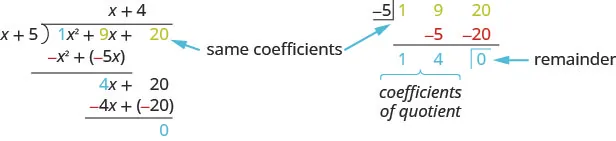Synthetic division basically just removes unnecessary repeated variables and numbers. Here all the $xx$ and $x2x2$ are removed. as well as the $−x2−x2$ and $−4x−4x$ as they are opposite the term above.

The first row of the synthetic division is the coefficients of the dividend. The $−5−5$ is the opposite of the 5 in the divisor.

The second row of the synthetic division are the numbers shown in red in the division problem.

The third row of the synthetic division are the numbers shown in blue in the division problem.

Notice the quotient and remainder are shown in the third row.

$Synthetic division only works when the divisor is of the formx−c.Synthetic division only works when the divisor is of the formx−c.$

The following example will explain the process.

### Example 5.42

Use synthetic division to find the quotient and remainder when $2x3+3x2+x+82x3+3x2+x+8$ is divided by $x+2.x+2.$

### Try It 5.83

Use synthetic division to find the quotient and remainder when $3x3+10x2+6x−23x3+10x2+6x−2$ is divided by $x+2.x+2.$

### Try It 5.84

Use synthetic division to find the quotient and remainder when $4x3+5x2−5x+34x3+5x2−5x+3$ is divided by $x+2.x+2.$

In the next example, we will do all the steps together.

### Example 5.43

Use synthetic division to find the quotient and remainder when $x4−16x2+3x+12x4−16x2+3x+12$ is divided by $x+4.x+4.$

### Try It 5.85

Use synthetic division to find the quotient and remainder when $x4−16x2+5x+20x4−16x2+5x+20$ is divided by $x+4.x+4.$

### Try It 5.86

Use synthetic division to find the quotient and remainder when $x4−9x2+2x+6x4−9x2+2x+6$ is divided by $x+3.x+3.$

### Divide Polynomial Functions

Just as polynomials can be divided, polynomial functions can also be divided.

### Division of Polynomial Functions

For functions $f(x)f(x)$ and $g(x),g(x),$ where $g(x)≠0,g(x)≠0,$

$(fg)(x)=f(x)g(x)(fg)(x)=f(x)g(x)$

### Example 5.44

For functions $f(x)=x2−5x−14f(x)=x2−5x−14$ and $g(x)=x+2,g(x)=x+2,$ find: $(fg)(x)(fg)(x)$ $(fg)(−4).(fg)(−4).$

### Try It 5.87

For functions $f(x)=x2−5x−24f(x)=x2−5x−24$ and $g(x)=x+3,g(x)=x+3,$ find $(fg)(x)(fg)(x)$ $(fg)(−3).(fg)(−3).$

### Try It 5.88

For functions $f(x)=x2−5x−36f(x)=x2−5x−36$ and $g(x)=x+4,g(x)=x+4,$ find $(fg)(x)(fg)(x)$ $(fg)(−5).(fg)(−5).$

### Use the Remainder and Factor Theorem

Let’s look at the division problems we have just worked that ended up with a remainder. They are summarized in the chart below. If we take the dividend from each division problem and use it to define a function, we get the functions shown in the chart. When the divisor is written as $x−c,x−c,$ the value of the function at $c,f(c),c,f(c),$ is the same as the remainder from the division problem.

Dividend Divisor $x−cx−c$ Remainder Function $f(c)f(c)$
$x4−x2+5x−6x4−x2+5x−6$ $x−(−2)x−(−2)$ $−4−4$ $f(x)=x4−x2+5x−6f(x)=x4−x2+5x−6$ $−4−4$
$3x3−2x2−10x+83x3−2x2−10x+8$ $x−2x−2$ 4 $f(x)=3x3−2x2−10x+8f(x)=3x3−2x2−10x+8$ 4
$x4−16x2+3x+15x4−16x2+3x+15$ $x−(−4)x−(−4)$ 3 $f(x)=x4−16x2+3x+15f(x)=x4−16x2+3x+15$ 3
Table 5.1

To see this more generally, we realize we can check a division problem by multiplying the quotient times the divisor and add the remainder. In function notation we could say, to get the dividend $f(x),f(x),$ we multiply the quotient, $q(x)q(x)$ times the divisor, $x−c,x−c,$ and add the remainder, r.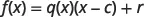If we evaluate this at $c,c,$ we get: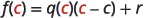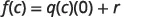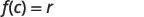This leads us to the Remainder Theorem.

### Remainder Theorem

If the polynomial function $f(x)f(x)$ is divided by $x−c,x−c,$ then the remainder is $f(c).f(c).$

### Example 5.45

Use the Remainder Theorem to find the remainder when $f(x)=x3+3x+19f(x)=x3+3x+19$ is divided by $x+2.x+2.$

### Try It 5.89

Use the Remainder Theorem to find the remainder when $f(x)=x3+4x+15f(x)=x3+4x+15$ is divided by $x+2.x+2.$

### Try It 5.90

Use the Remainder Theorem to find the remainder when $f(x)=x3−7x+12f(x)=x3−7x+12$ is divided by $x+3.x+3.$

When we divided $8a3+278a3+27$ by $2a+32a+3$ in Example 5.41 the result was $4a2−6a+9.4a2−6a+9.$ To check our work, we multiply $4a2−6a+94a2−6a+9$ by $2a+32a+3$ to get $8a3+278a3+27$.

$(4a2−6a+9)(2a+3)=8a3+27(4a2−6a+9)(2a+3)=8a3+27$

Written this way, we can see that $4a2−6a+94a2−6a+9$ and $2a+32a+3$ are factors of $8a3+27.8a3+27.$ When we did the division, the remainder was zero.

Whenever a divisor, $x−c,x−c,$ divides a polynomial function, $f(x),f(x),$ and resulting in a remainder of zero, we say $x−cx−c$ is a factor of $f(x).f(x).$

The reverse is also true. If $x−cx−c$ is a factor of $f(x)f(x)$ then $x−cx−c$ will divide the polynomial function resulting in a remainder of zero.

We will state this in the Factor Theorem.

### Factor Theorem

For any polynomial function $f(x),f(x),$

• if $x−cx−c$ is a factor of $f(x),f(x),$ then $f(c)=0f(c)=0$
• if $f(c)=0,f(c)=0,$ then $x−cx−c$ is a factor of $f(x)f(x)$

### Example 5.46

Use the Remainder Theorem to determine if $x−4x−4$ is a factor of $f(x)=x3−64.f(x)=x3−64.$

### Try It 5.91

Use the Factor Theorem to determine if $x−5x−5$ is a factor of $f(x)=x3−125.f(x)=x3−125.$

### Try It 5.92

Use the Factor Theorem to determine if $x−6x−6$ is a factor of $f(x)=x3−216.f(x)=x3−216.$

### Media

Access these online resources for additional instruction and practice with dividing polynomials.

### Section 5.4 Exercises

#### Practice Makes Perfect

Divide Monomials

In the following exercises, divide the monomials.

288.

$15 r 4 s 9 ÷ ( 15 r 4 s 9 ) 15 r 4 s 9 ÷ ( 15 r 4 s 9 )$

289.

$20 m 8 n 4 ÷ ( 30 m 5 n 9 ) 20 m 8 n 4 ÷ ( 30 m 5 n 9 )$

290.

$18 a 4 b 8 −27 a 9 b 5 18 a 4 b 8 −27 a 9 b 5$

291.

$45 x 5 y 9 −60 x 8 y 6 45 x 5 y 9 −60 x 8 y 6$

292.

$( 10 m 5 n 4 ) ( 5 m 3 n 6 ) 25 m 7 n 5 ( 10 m 5 n 4 ) ( 5 m 3 n 6 ) 25 m 7 n 5$

293.

$( −18 p 4 q 7 ) ( −6 p 3 q 8 ) −36 p 12 q 10 ( −18 p 4 q 7 ) ( −6 p 3 q 8 ) −36 p 12 q 10$

294.

$( 6 a 4 b 3 ) ( 4 a b 5 ) ( 12 a 2 b ) ( a 3 b ) ( 6 a 4 b 3 ) ( 4 a b 5 ) ( 12 a 2 b ) ( a 3 b )$

295.

$( 4 u 2 v 5 ) ( 15 u 3 v ) ( 12 u 3 v ) ( u 4 v ) ( 4 u 2 v 5 ) ( 15 u 3 v ) ( 12 u 3 v ) ( u 4 v )$

Divide a Polynomial by a Monomial

In the following exercises, divide each polynomial by the monomial.

296.

$( 9 n 4 + 6 n 3 ) ÷ 3 n ( 9 n 4 + 6 n 3 ) ÷ 3 n$

297.

$( 8 x 3 + 6 x 2 ) ÷ 2 x ( 8 x 3 + 6 x 2 ) ÷ 2 x$

298.

$( 63 m 4 − 42 m 3 ) ÷ ( −7 m 2 ) ( 63 m 4 − 42 m 3 ) ÷ ( −7 m 2 )$

299.

$( 48 y 4 − 24 y 3 ) ÷ ( −8 y 2 ) ( 48 y 4 − 24 y 3 ) ÷ ( −8 y 2 )$

300.

$66 x 3 y 2 − 110 x 2 y 3 − 44 x 4 y 3 11 x 2 y 2 66 x 3 y 2 − 110 x 2 y 3 − 44 x 4 y 3 11 x 2 y 2$

301.

$72 r 5 s 2 + 132 r 4 s 3 − 96 r 3 s 5 12 r 2 s 2 72 r 5 s 2 + 132 r 4 s 3 − 96 r 3 s 5 12 r 2 s 2$

302.

$10 x 2 + 5 x − 4 −5 x 10 x 2 + 5 x − 4 −5 x$

303.

$20 y 2 + 12 y − 1 −4 y 20 y 2 + 12 y − 1 −4 y$

Divide Polynomials using Long Division

In the following exercises, divide each polynomial by the binomial.

304.

$( y 2 + 7 y + 12 ) ÷ ( y + 3 ) ( y 2 + 7 y + 12 ) ÷ ( y + 3 )$

305.

$( a 2 − 2 a − 35 ) ÷ ( a + 5 ) ( a 2 − 2 a − 35 ) ÷ ( a + 5 )$

306.

$( 6 m 2 − 19 m − 20 ) ÷ ( m − 4 ) ( 6 m 2 − 19 m − 20 ) ÷ ( m − 4 )$

307.

$( 4 x 2 − 17 x − 15 ) ÷ ( x − 5 ) ( 4 x 2 − 17 x − 15 ) ÷ ( x − 5 )$

308.

$( q 2 + 2 q + 20 ) ÷ ( q + 6 ) ( q 2 + 2 q + 20 ) ÷ ( q + 6 )$

309.

$( p 2 + 11 p + 16 ) ÷ ( p + 8 ) ( p 2 + 11 p + 16 ) ÷ ( p + 8 )$

310.

$( 3 b 3 + b 2 + 4 ) ÷ ( b + 1 ) ( 3 b 3 + b 2 + 4 ) ÷ ( b + 1 )$

311.

$( 2 n 3 − 10 n + 28 ) ÷ ( n + 3 ) ( 2 n 3 − 10 n + 28 ) ÷ ( n + 3 )$

312.

$( z 3 + 1 ) ÷ ( z + 1 ) ( z 3 + 1 ) ÷ ( z + 1 )$

313.

$( m 3 + 1000 ) ÷ ( m + 10 ) ( m 3 + 1000 ) ÷ ( m + 10 )$

314.

$( 64 x 3 − 27 ) ÷ ( 4 x − 3 ) ( 64 x 3 − 27 ) ÷ ( 4 x − 3 )$

315.

$( 125 y 3 − 64 ) ÷ ( 5 y − 4 ) ( 125 y 3 − 64 ) ÷ ( 5 y − 4 )$

Divide Polynomials using Synthetic Division

In the following exercises, use synthetic Division to find the quotient and remainder.

316.

$x3−6x2+5x+14x3−6x2+5x+14$ is divided by $x+1x+1$

317.

$x3−3x2−4x+12x3−3x2−4x+12$ is divided by $x+2x+2$

318.

$2x3−11x2+11x+122x3−11x2+11x+12$ is divided by $x−3x−3$

319.

$2x3−11x2+16x−122x3−11x2+16x−12$ is divided by $x−4x−4$

320.

$x4−5x2+13x+3x4−5x2+13x+3$ is divided by $x+3x+3$

321.

$x4+x2+6x−10x4+x2+6x−10$ is divided by $x+2x+2$

322.

$2x4−9x3+5x2−3x−62x4−9x3+5x2−3x−6$ is divided by $x−4x−4$

323.

$3x4−11x3+2x2+10x+63x4−11x3+2x2+10x+6$ is divided by $x−3x−3$

Divide Polynomial Functions

In the following exercises, divide.

324.

For functions $f(x)=x2−13x+36f(x)=x2−13x+36$ and $g(x)=x−4,g(x)=x−4,$ find $(fg)(x)(fg)(x)$ $(fg)(−1)(fg)(−1)$

325.

For functions $f(x)=x2−15x+54f(x)=x2−15x+54$ and $g(x)=x−9,g(x)=x−9,$ find $(fg)(x)(fg)(x)$ $(fg)(−5)(fg)(−5)$

326.

For functions $f(x)=x3+x2−7x+2f(x)=x3+x2−7x+2$ and $g(x)=x−2,g(x)=x−2,$ find $(fg)(x)(fg)(x)$ $(fg)(2)(fg)(2)$

327.

For functions $f(x)=x3+2x2−19x+12f(x)=x3+2x2−19x+12$ and $g(x)=x−3,g(x)=x−3,$ find $(fg)(x)(fg)(x)$ $(fg)(0)(fg)(0)$

328.

For functions $f(x)=x2−3x+2f(x)=x2−3x+2$ and $g(x)=x+3,g(x)=x+3,$ find $(fg)(x)(fg)(x)$ $(fg)(3)(fg)(3)$

329.

For functions $f(x)=x2+2x−3f(x)=x2+2x−3$ and $g(x)=x+3g(x)=x+3$ find $(fg)(x)(fg)(x)$ $(fg)(3)(fg)(3)$

Use the Remainder and Factor Theorem

In the following exercises, use the Remainder Theorem to find the remainder.

330.

$f(x)=x3−8x+7f(x)=x3−8x+7$ is divided by $x+3x+3$

331.

$f(x)=x3−4x−9f(x)=x3−4x−9$ is divided by $x+2x+2$

332.

$f(x)=2x3−6x−24f(x)=2x3−6x−24$ divided by $x−3x−3$

333.

$f(x)=7x2−5x−8f(x)=7x2−5x−8$ divided by $x−1x−1$

In the following exercises, use the Factor Theorem to determine if $x−cx−c$ is a factor of the polynomial function.

334.

Determine whether $x+3x+3$ a factor of $x3+8x2+21x+18x3+8x2+21x+18$

335.

Determine whether $x+4x+4$ a factor of $x3+x2−14x+8x3+x2−14x+8$

336.

Determine whether $x−2x−2$ a factor of $x3−7x2+7x−6x3−7x2+7x−6$

337.

Determine whether $x−3x−3$ a factor of $x3−7x2+11x+3x3−7x2+11x+3$

#### Writing Exercises

338.

James divides $48y+648y+6$ by 6 this way: $48y+66=48y.48y+66=48y.$ What is wrong with his reasoning?

339.

Divide $10x2+x−122x10x2+x−122x$ and explain with words how you get each term of the quotient.

340.

Explain when you can use synthetic division.

341.

In your own words, write the steps for synthetic division for $x2+5x+6x2+5x+6$ divided by $x−2.x−2.$

#### Self Check

After completing the exercises, use this checklist to evaluate your mastery of the objectives of this section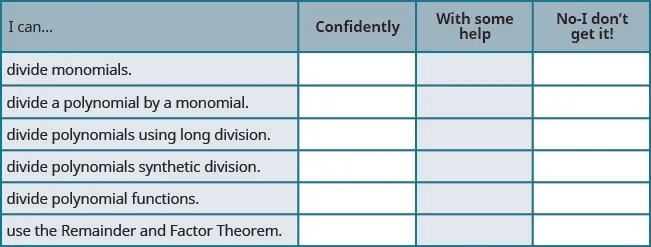On a scale of 1-10, how would you rate your mastery of this section in light of your responses on the checklist? How can you improve this?

Order a print copy

As an Amazon Associate we earn from qualifying purchases.